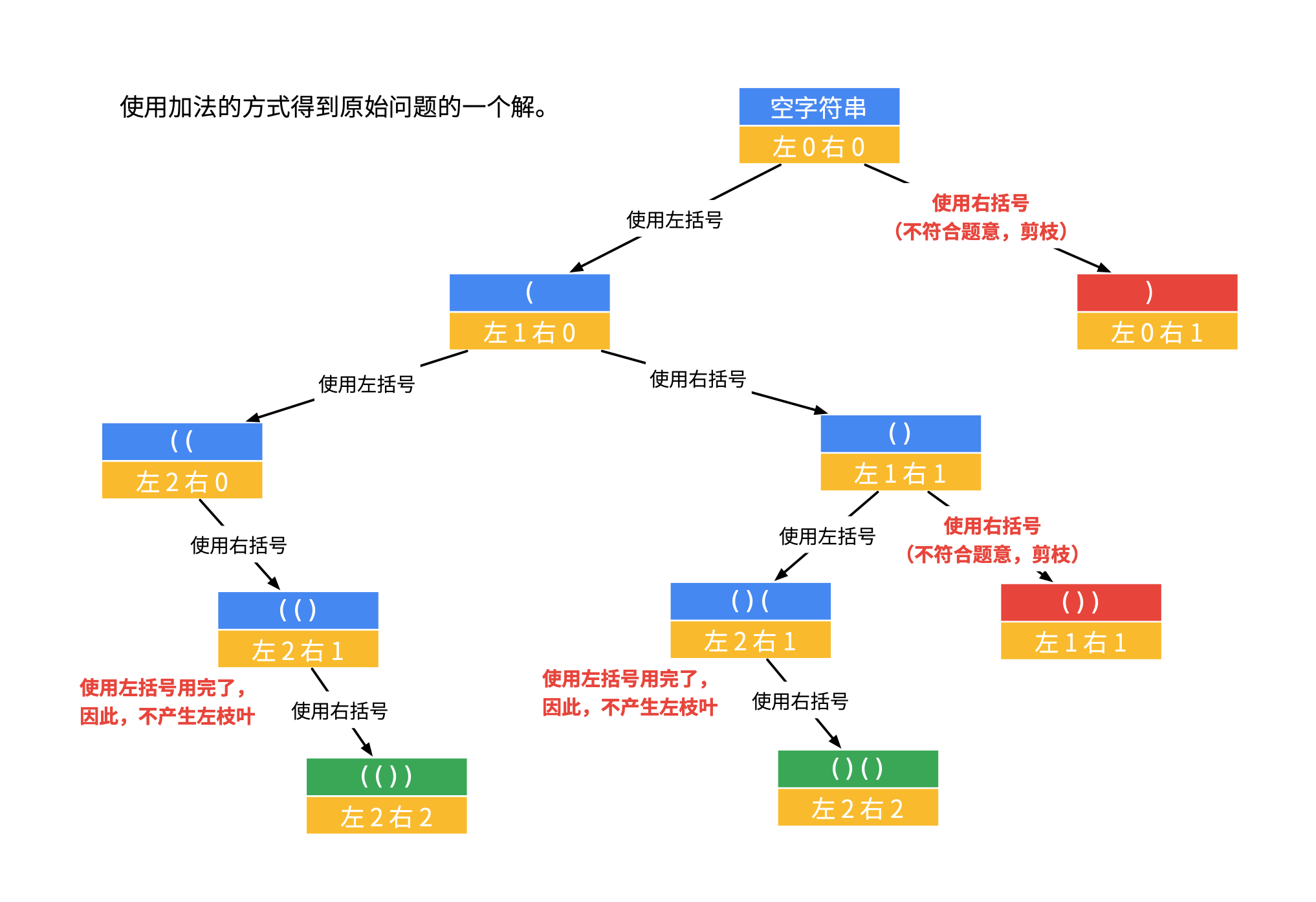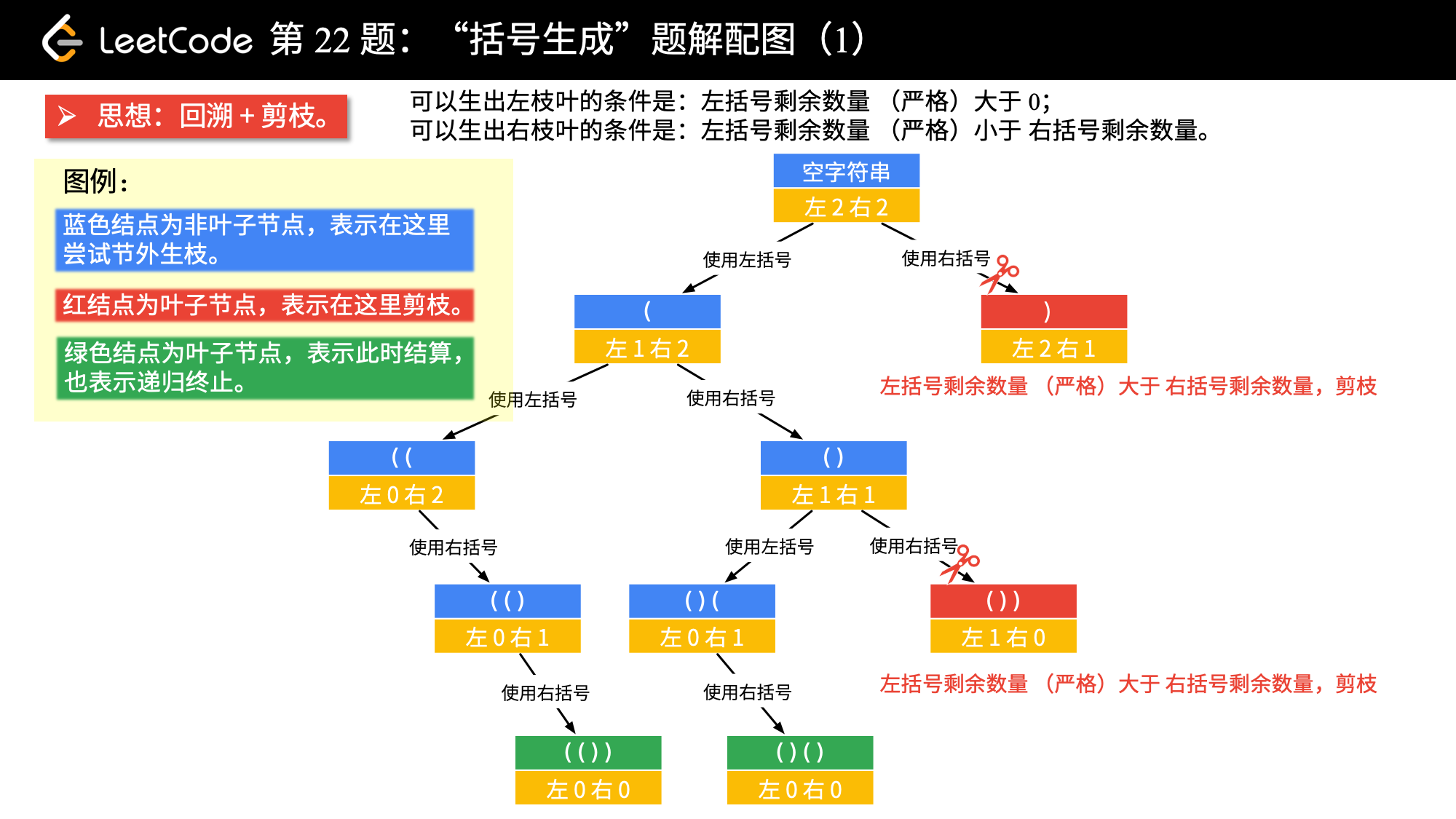## 括号生成

### 题目

输入：n = 3

"((()))",
"(()())",
"(())()",
"()(())",
"()()()"
]


## 思路1-暴力破万法

### 流程

1. 构建所有 () 可能构成的字符串

2. 过滤出其中符合条件的字符串。

### 示例代码

/**
*
* 思路：
*
* （1）生成所有可能
* （2）如果符合则加入结果
*
* @param n 数字
* @return 结果
*/
public List<String> generateParenthesis(int n) {
List<String> all = generateAll(n);
List<String> resultList = new ArrayList<>();
for(String string : all) {
if(isValid(string)) {
}
}
return resultList;
}

/**
* 生成所有的可能字符串
* @param n 个数
* @return 结果
*/
private List<String> generateAll(final int n) {
List<String> resultList = new ArrayList<>();
char[] chars = new char[]{'(', ')'};
for(int i = 0; i < n*2; i++) {
List<String> newList = new ArrayList<>();
for(String result : resultList) {
for(char c: chars) {
}
}
resultList = newList;
}
return resultList;
}

/**
* 是否合法
* @param s 字符串
* @return 结果
*/
private boolean isValid(final String s) {
int length = s.length();
int headIx = 0;
for(int i = 0; i < length; i++) {
char c = s.charAt(i);
if('(' == c) {
} else {
if(headIx == 0) {
return false;
}
}
}
return headIx == 0;
}


## 思路2-DFS 深度优先遍历### 流程

1. 第一个有效符号一定是 (

2. ( 个数比 ) 少的时候，进行剪枝

3. () 的个数都等于 n 的时候，一次遍历就结束了。

### 实现

public List<String> generateParenthesis(int n) {
List<String> resultList = new ArrayList<>();
dfs(resultList, "", 0, 0, n);
return resultList;
}

/**
* 深度优先遍历
* @param resultList 结果列表
* @param string 字符串
* @param left 左括号
* @param right 右括号
* @param num 位数
*/
private void dfs(List<String> resultList,
String string,
int left,
int right,
int num) {
if(left == num && right == num) {
return;
}
//左边的括号是可以一直加的
if(left < num) {
dfs(resultList, string+"(", left+1, right, num);
}
// 这里是对剪枝处理的优化，如果 left < right，是跳过的。
// ) 小于 < 的时候，可以添加
if(right < left) {
dfs(resultList, string+")", left, right+1, num);
}
}


### 性能

Runtime: 1 ms, faster than 85.42% of Java online submissions for Generate Parentheses.
Memory Usage: 39 MB, less than 98.86% of Java online submissions for Generate Parentheses.


## 思路3-DFS 深度优先遍历改进

### 实现

public List<String> generateParenthesis(int n) {
List<String> resultList = new ArrayList<>();
StringBuilder stringBuilder = new StringBuilder(n << 1);
backtrack(resultList, stringBuilder, 0, 0, n);
return resultList;
}

/**
* 递归处理
* @param resultList 结果列表
* @param stringBuilder 字符串
* @param left 左括号
* @param right 右括号
* @param num 位数
*/
private void backtrack(List<String> resultList,
StringBuilder stringBuilder,
int left,
int right,
int num) {
if(stringBuilder.length() == num << 1) {
return;
}
//左边的括号是可以一直加的
if(left < num) {
backtrack(resultList, stringBuilder.append("("), left+1, right, num);
// 重新设置
stringBuilder.setLength(stringBuilder.length()-1);
}
// ) 小于 < 的时候，可以添加
if(right < left) {
backtrack(resultList, stringBuilder.append(")"), left, right+1, num);
stringBuilder.setLength(stringBuilder.length()-1);
}
}


### 性能

Runtime: 0 ms, faster than 100.00% of Java online submissions for Generate Parentheses.
Memory Usage: 39.6 MB, less than 63.29% of Java online submissions for Generate Parentheses.


## 思路4-DFS 深度优先方式2### java 实现

public List<String> generateParenthesis(int n) {
List<String> resultList = new ArrayList<>();
dfs(resultList, "", n, n, n);
return resultList;
}

/**
* 深度优先遍历
* @param resultList 结果列表
* @param string 字符串
* @param left 左括号
* @param right 右括号
* @param num 位数
*/
private void dfs(List<String> resultList,
String string,
int left,
int right,
int num) {
if(left == 0 && right == 0) {
return;
}
// 剪枝（左括号可以使用的个数严格大于右括号可以使用的个数）
if (left > right) {
return;
}
if(left > 0) {
dfs(resultList, string+"(", left-1, right, num);
}
if(right > 0) {
dfs(resultList, string+")", left, right-1, num);
}
}


## 思路5-BFS 广度优先遍历

### java 实现

class Node {
private String text;
private int left;
private int right;

public Node(String text, int left, int right) {
this.text = text;
this.left = left;
this.right = right;
}
}

/**
* @param n 数字
* @return 结果
*/
public List<String> generateParenthesis(int n) {
List<String> res = new ArrayList<>();
if (n == 0) {
return res;
}
// 从上到下
Queue<Node> queue = new LinkedList<>();
Node rootNode = new Node("", 0, 0);
while (!queue.isEmpty()) {
Node curNode = queue.remove();
// 最后一层
if (curNode.left == n && curNode.right == n) {
}
if (curNode.left < n) {
queue.add(new Node(curNode.text + "(", curNode.left + 1, curNode.right));
}
// 剪枝
if (curNode.right < curNode.left) {
queue.add(new Node(curNode.text + ")", curNode.left, curNode.right + 1));
}
}
return res;
}


## 思路6-DP 动态规划

### 核心思路

1. 我们需要枚举与第一个 ( 对应的 ) 的位置 2 * i + 1；

2. 递归调用 generate(i) 即可计算 a 的所有可能性；

3. 递归调用 generate(n - i - 1) 即可计算 b 的所有可能性；

4. 遍历 a 与 b 的所有可能性并拼接，即可得到所有长度为 2 * n 的括号序列。

ps: DP 最核心的两点

（1）找到递推公式

（2）缓存结果，避免重复计算

### java 实现

public List<String> generateParenthesis(int n) {
// 存放缓存信息
List<List<String>> cache = new ArrayList<>();
// 初始化第一个元素列表为 [""]
for (int i = 1; i <= n; i++) {
List<String> cur = new ArrayList<>();
for (int j = 0; j < i; j++) {
List<String> str1 = cache.get(j);
List<String> str2 = cache.get(i - 1 - j);
for (String s1 : str1) {
for (String s2 : str2) {
// 枚举右括号的位置
cur.add("(" + s1 + ")" + s2);
}
}
}
}
return cache.get(n);
}


### 性能

Runtime: 7 ms, faster than 13.20% of Java online submissions for Generate Parentheses.
Memory Usage: 39.5 MB, less than 65.23% of Java online submissions for Generate Parentheses.


## 参考资料

generate-parentheses